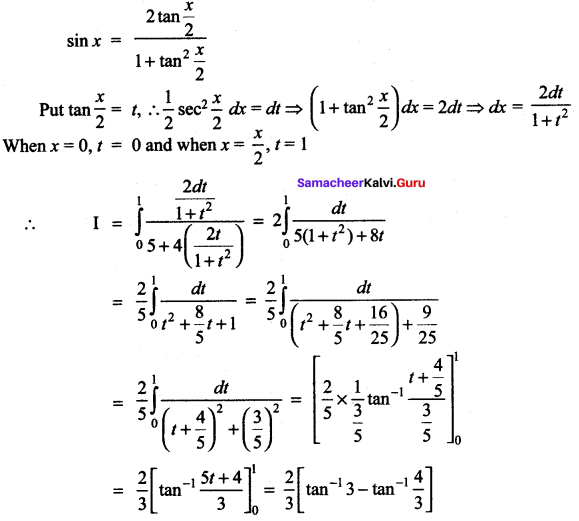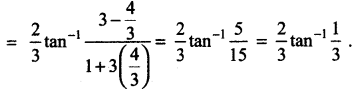## Tamilnadu Samacheer Kalvi 12th Maths Solutions Chapter 9 Applications of Integration Ex 9.5

Evaluate the following:

Question 1.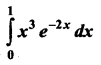Solution:
u = x3 ; dv = e-2
Applying Bernoulli’s formula, we get,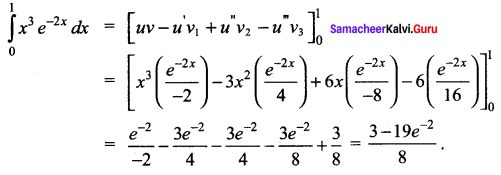Question 2.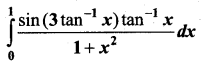Solution: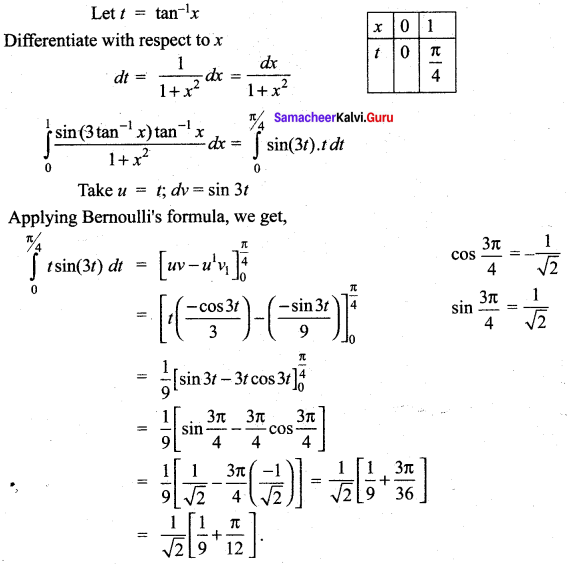Question 3.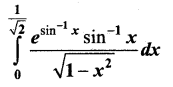Solution: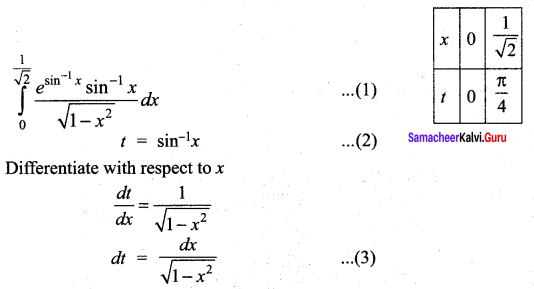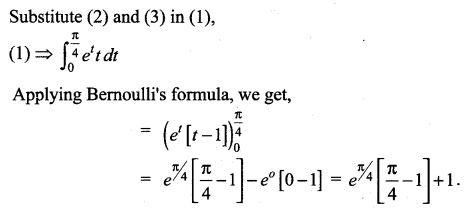Question 4.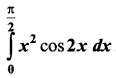Solution: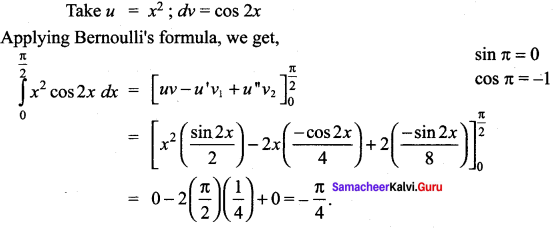### Samacheer Kalvi 12th Maths Solutions Chapter 9 Applications of Integration Ex 9.4 Additional Problems

Question 1.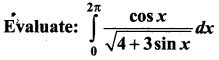Solution: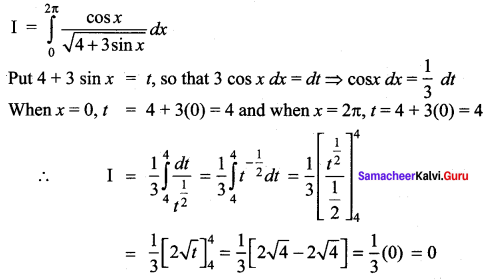Question 2.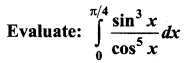Solution: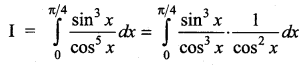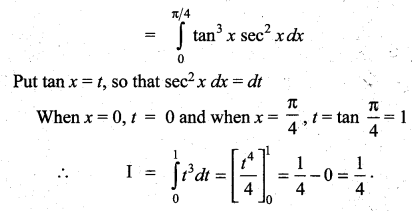Question 3.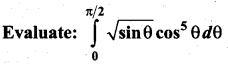Solution: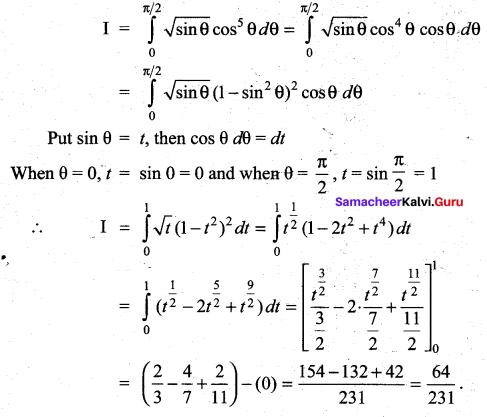Question 4.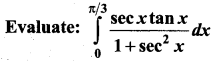Solution: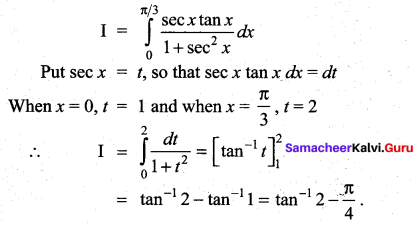Question 5.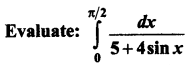Solution: